Related Articles

# Class 10 RD Sharma Solutions – Chapter 4 Triangles – Exercise 4.5 | Set 1

• Last Updated : 30 Apr, 2021

### Question 1. In fig., ΔACB ∼ ΔAPQ. If BC = 8 cm, PQ = 4 cm, BA = 6.5 cm and AP = 2.8 cm, find CA and AQ.

Solution: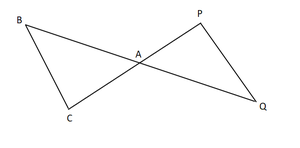Attention reader! All those who say programming isn't for kids, just haven't met the right mentors yet. Join the  Demo Class for First Step to Coding Coursespecifically designed for students of class 8 to 12.

The students will get to learn more about the world of programming in these free classes which will definitely help them in making a wise career choice in the future.

Given,

ΔACB ∼ ΔAPQ

BC = 8 cm, PQ = 4 cm, BA = 6.5 cm and AP = 2.8 cm

To find: CA and AQ

We know that,

ΔACB ∼ ΔAPQ             [given]BA/ AQ = CA/ AP = BC/ PQ       [sides are proportional of Similar Triangles]

Now,

6.5/ AQ = 8/ 4

AQ = (6.5 x 4)/ 8

AQ = 3.25 cm

Similarly,

CA/ AP = BC/ PQ

CA/ 2.8 = 8/ 4

CA = 2.8 × 2

CA = 5.6 cm

Hence, CA = 5.6 cm and AQ = 3.25 cm.

### Question 2. In fig., AB ∥ QR, find the length of PB.

Solution: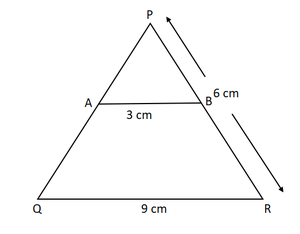Given,

ΔPQR, AB ∥ QR

AB = 3 cm, QR = 9 cm and PR = 6 cm

To find: length of PB

In ΔPAB and ΔPQR

We have,

∠P = ∠P            [Common angle ]

∠PAB = ∠PQR       [Corresponding angles]

∠PBA = ∠PRQ        [Corresponding angles]ΔPAB ∼ ΔPQR          [By AAA similarity criteria]AB/ QR = PB/ PR     [sides are proportional of Similar Triangles]

⇒ 3/ 9 = PB/6

PB = 6/3

Hence the length of PB = 2 cm

### Question 3. In fig. given, XY∥BC. Find the length of XY.

Solution: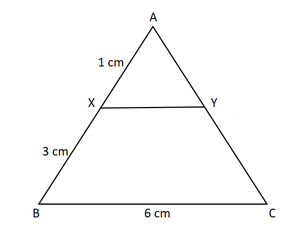Given,

XY∥BC

AX = 1 cm, XB = 3 cm and BC = 6 cm

To find: length of XY

In ΔAXY and ΔABC

We have,

∠A = ∠A             [Common angle]

∠AXY = ∠ABC    [Corresponding angles]

∠AYX = ∠ACB  [Corresponding angles]ΔAXY ∼ ΔABC    [By AAA similarity criteria]XY/ BC = AX/ AB   [Corresponding Parts of Similar Triangles are propositional]

Now,

(AB = AX + XB = 1 + 3 = 4)

XY/6 = 1/4

XY/1 = 6/4

Hence, length of XY = 1.5 cm

### Question 4. In a right-angled triangle with sides a and b and hypotenuse c, the altitude drawn on the hypotenuse is x. Prove that ab = cx.

Solution: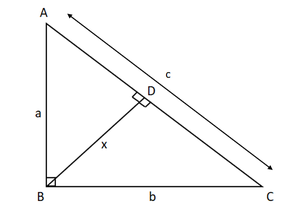Let us consider  ΔABC to be a right angle triangle with sides a,b and c as  hypotenuse. let BD be the altitude drawn on the hypotenuse AC.

To prove: ab = cx

Now,

∠BAC = ∠DAB    [Common]

∠ACB = ∠ABC = 90[right angled triangle]ΔABC ∼ ΔADB              [By AA similarity criteria]

So,

AC/ AB = BC/ EB      [Corresponding Parts of Similar Triangles are propositional]

c/ a = b/ x

⇒ xc = abab = cx

Hence, proved.

### Question 5. In fig., ∠ABC = 90° and BD⊥AC. If BD = 8 cm, and AD = 4 cm, find CD.

Solution: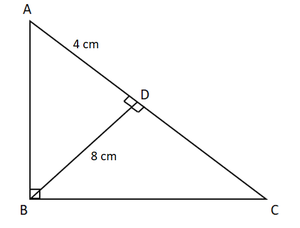Given,

ABC is a right-angled triangle and BD⊥AC.

BD = 8 cm, and AD = 4 cm

To find: CD.

Now in triangle ΔABD and ΔCBD,

∠BDC=∠BDA        [each 90]

∠ABD=∠CBD      [BD⊥AC]ΔDBA∼ΔDCB  [By AA similarity]

(8)2 = 4 x DC

DC = 64/4 = 16 cm

Hence, the length of side CD = 16 cm

### Question 6. In fig., ∠ABC = 90o and BD ⊥ AC. If AB = 5.7 cm, BD = 3.8 cm and CD = 5.4 cm, Find BC.

Solution: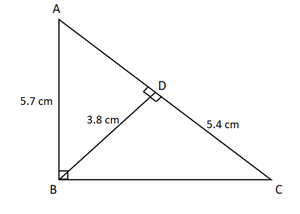Given:

BD ⊥ AC

AB = 5.7 cm, BD = 3.8 cm and CD = 5.4 cm

∠ABC = 90o

Required to find: BC

let ∠BCD=x

∠ADB = ∠CDB = 90o [right angled triangle]

∠ABD = ∠CBD       [∠ABD = ∠CBD = 90o – x]ΔABC ∼ ΔBDC [By AA similarity]

so,

AB/ BD = BC/ CD     [Corresponding Parts of Similar Triangles are propositional]5.7/ 3.8 = BC/ 5.4

BC = (5.7 × 5.4)/ 3.8 = 8.1

Hence, length of side BC = 8.1 cm

### Question 7. In the fig. given, DE ∥ BC such that AE = (1/4)AC. If AB = 6 cm, find AD.

Solution: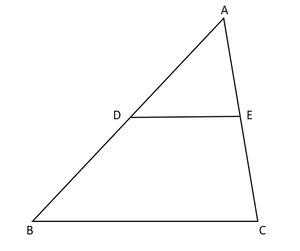Given:

DE∥BC

AE = (1/4)AC

AB = 6 cm.

∠A = ∠A        [Common angle]ΔADE ∼ ΔABC   [By AA similarity criteria]

so,AD/AB = AE/ AC   [Corresponding Parts of Similar Triangles are propositional]AD=1.5 cm

Hence length of AD = 1.5 cm

### Question 8. In the fig. given, if AB ⊥ BC, DC ⊥ BC, and DE ⊥ AC, prove that ΔCED ∼ ΔABC

Solution: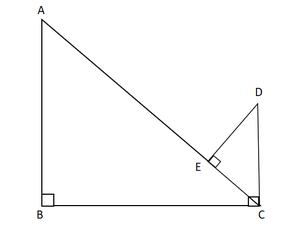Given:

AB ⊥ BC,

DC ⊥ BC,

DE ⊥ AC

To prove: ΔCED∼ΔABC

Now In ΔABC and ΔCED,

∠B = ∠E = 90o   [given]

∠BAC = ∠ECD     [alternate angles]ΔCED∼ΔABC  [AA similarity]

Hence Proved

### Question 9. Diagonals AC and BD of a trapezium ABCD with AB ∥ DC intersect each other at the point O. Using similarity criterion for two triangles, show that OA/ OC = OB/ OD

Solution: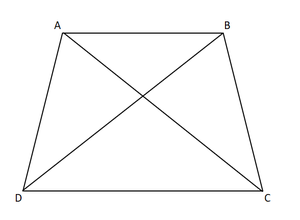Given: OC is the point of intersection of AC and BD in the trapezium ABCD, with AB ∥ DC.

To prove: OA/ OC = OB/ OD

Now In ΔAOB and ΔCOD,

∠AOB = ∠COD [Vertically Opposite Angles]

∠OAB = ∠OCD    [Alternate angles]ΔAOB ∼ ΔCOD

so,OA/ OC = OB/ OD   [Corresponding sides are proportional]

Hence Proved

### Question 10. If Δ ABC and Δ AMP are two right triangles, right angled at B and M, respectively such that ∠MAP = ∠BAC. Prove that

(i) ΔABC ∼ ΔAMP

(ii) CA/ PA = BC/ MP

Solution: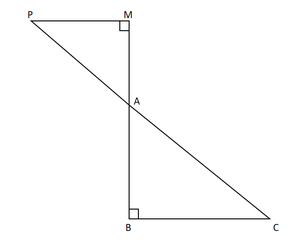(i)  Given:

Δ ABC and Δ AMP are the two right triangles.

Now In ΔABC and ΔAMP,

∠AMP = ∠B = 90o

∠MAP = ∠BAC   [Vertically Opposite Angles]ΔABC∼ΔAMP     [AA similarity]

(ii) Since, ΔABC∼ΔAMP

CA/ PA = BC/ MP  [Corresponding sides are proportional]

Hence, proved.

### Question 11. A vertical stick 10 cm long casts a shadow 8 cm long. At the same time, a tower casts a shadow 30 m long. Determine the height of the tower.

Solution: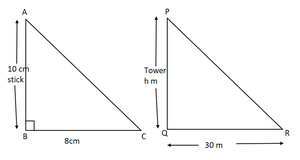Given:

Length of stick = 10cm

Length of the stick’s shadow = 8cm

Length of the tower’s shadow = 30m = 3000cm

To find: the height of the tower = PQ.

Now In ΔABC and ΔPQR,

∠ABC = ∠PQR = 90 [each 90o]

∠ACB = ∠PRQ   [angle are made at the same time]ΔABC ∼ ΔPQR    [By AA similarity]

So,

AB/BC = PQ/QR    [by Corresponding sides are proportional]

10/8 = PQ/ 3000

PQ = (3000×10)/ 8

PQ = 30000/8

PQ = 3750/100

Hence the length of PQ = 37.5 m

### Question 12. In fig., ∠A = ∠CED, prove that ΔCAB ∼ ΔCED. Also, find the value of x.

Solution: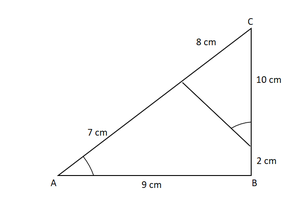Given:

∠A = ∠CED

To prove: ΔCAB ∼ ΔCED

In ΔCAB and ΔCED

∠C = ∠C  [Common]

∠A = ∠CED [Given]ΔCAB ∼ ΔCED [By AA similarity]

so,

CA/ CE = AB/ ED  [by Corresponding sides are proportional]

15/10 = 9/x

x = (9 × 10)/ 15

Hence, value for x = 6 cm

My Personal Notes arrow_drop_up